# Design of Raft Foundations – Methods and CalculationsReading time: 1 minute

## Design of Raft Foundations - Methods and Calculations

According to IS – 2950:1965, the design criteria of raft footings are given below: The maximum differential settlement in foundation on clayey soils and sandy soils should not exceed 40mm and 25mm respectively. The maximum settlement should generally be limited to the following values:
• Raft foundation on clay – 65 to 100 mm.
• Raft foundation on sand – 40 to 65 mm.There are two methods for the design of raft foundations. They are:
1. Conventional Method
2. Soil Line Method.

### 1. Conventional Method of Raft Foundation Design

#### Assumptions:

1. The soil pressure is assumed to be plane such that the centroid of the soil pressure coincides with the line of action of the resultant force of all the loads acting on the foundation. 2. The foundation is infinitely rigid and therefore, the actual deflection of the raft does not influence the pressure distribution below the raft. In this method, allowable bearing pressure can be calculated by the following formulae: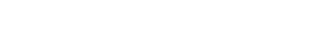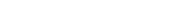Where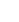and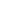= allowable soil pressure under raft foundation in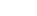(use a factor of safety of three). The smaller values ofandshould be used for design.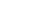and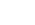= reduction factor on account of subsoil water. N = penetration resistance. If the values of N is greater than 15 in saturated silts, the equivalent penetration resistance should be taken for the design. The equivalent penetration resistance can be determined by the formula: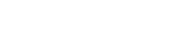The pressure distribution (q) under the raft should be calculated by the following formula: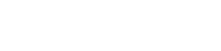Where Q = total vertical load on raft x, y = co-ordinates of any given point on the raft with respect to the x and y axes passing through the centroid of the area of the raft. A = total area of the raft.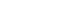= eccentricities about the principal axis passing through the centroid of the section.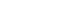= moment of inertia about the principal axis through the centroid of the section.,can be calculated by the following equations: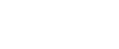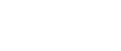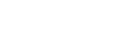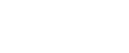Where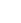and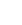= eccentricities in x and y direction of the load from the centroid.and= moment of inertia of the area of the raft respectively about the x and y axes through the centroid.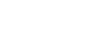for the whole area about x and y axes through the centroid.

### 2) Soil line Method (Elastic Method) of Raft Foundation Design

A number of methods have been proposed based on primarily on two approaches of simplified and truly elastic foundations. i. Simplified elastic foundation: The soil in this method is replaced by an infinite number of isolated springs. ii. Truly elastic foundation: The soil is assumed to be continuous elastic medium obeying Hooke’s law. In the case of foundation which is comparatively flexible and where loads tend to concentrate over small areas these methods are to be used. The method assumes in addition to other factors that the modulus of subgrade reaction, determined from tests is known. The modulus of subgrade reaction (Ks) as applicable to the case of load through a plate of size 30 cm x 30 cm or beams 20 cm wide on soil area is given in table-1 for cohesionless soils and table-2 for cohesive soils. Table -1: Modulus of subgrade reaction Ks for cohesionless soils
 Soil Characteristics Ks(Kg/cm2) Relative Density Values of N Dry or moist state Submerged state 1. Loose <10 1.5 0.9 1. Medium 10 to <30 4.7 2.9 3. Dense 30 and over 18 10.8
Table – 2: Modulus of subgrade reaction Kfor cohesive soils
 Soil Characteristics Ks(Kg/cm2) Consistency Unconfined compressive Strength (Kg/cm2)) - 1. Stiff 1 to <2 2.7 1. Very Stiff 2 to <4 5.4 3. Hard 4 and over 10.8
The above values of Ks are corresponding to a square plate of size 30 cm x 30 cm. To find the values of K corresponding to different sizes and shapes, the following relationships to be used. (a) Effect of size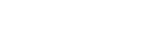for cohesionless soil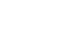for cohesive soils. Where, K = modulus of subgrade reaction for footing of width B cm Ks = modulus of subgrade reaction for a square plate of width 30cm x 30cm K’ = modulus of subgrade reaction for footing of width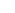cm. (b) Effect of shape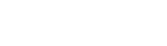for cohesive soils Where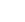= modulus of subgrade reaction for a rectangular footing having length L and width B.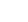= modulus of subgrade reaction for square footing of side B. The effect of shape is negligible in the case of footing on cohesionless soils.